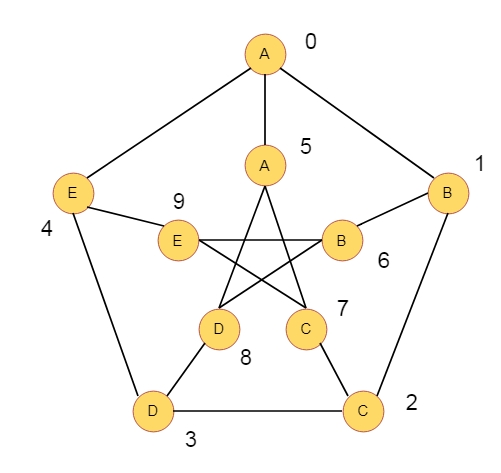# A Peterson Graph Problem in C Program?

CServer Side ProgrammingProgramming

Suppose we have one graph like below. That graph is Peterson graph. The vertices are numbered from 0 through 9. Each vertex has some letters. Let consider one walk W, in that graph, where L vertices are used. A string S with L letters is realized by the walk W when the letter sequence in W and S are same. We can visit the vertices multiple times.For example, one string S is like “ABBECCD”, this is realized by the walk (0, 1, 6, 9, 7, 2, 3). Our task is to find such walk, and if that walk is present, then find lexicographically least such walk. If no suck walk is there, then return -1.

## Algorithm

petersonGraphWalk(S, v) −

begin
res := starting vertex
for each character c in S except the first one, do
if there is an edge between v and c in outer graph, then
v := c
else if there is an edge between v and c+5 in inner graph, then
v := c + 5
else
return false
end if
put v into res
done
return true
end

## Example

#include<iostream>
using namespace std;
bool adj_mat = {{0, 1, 0, 0, 1, 1, 0, 0, 0, 0},
{1, 0, 1, 0, 0, 0, 1, 0, 0, 0},
{0, 1, 0, 1, 0, 0, 0, 1, 0, 0},
{0, 0, 1, 0, 1, 0, 0, 0, 1, 0},
{1, 0, 0, 1, 0, 0, 0, 0, 0, 1},
{1, 0, 0, 0, 0, 0, 0, 1, 1, 0},
{0, 1, 0, 0, 0, 0, 0, 0, 1, 1},
{0, 0, 1, 0, 0, 1, 0, 0, 0, 1},
{0, 0, 0, 1, 0, 1, 1, 0, 0, 0},
{0, 0, 0, 0, 1, 0, 1, 1, 0, 0}
};
char S;
char res;
bool petersonGraphWalk(char* S, int v){
res = v + '0';
for(int i = 1; S[i]; i++){
//traverse the outer graph
v = S[i] - 'A';
}
//then check the inner graph
else if(adj_mat[v][S[i] - 'A' + 5] || adj_mat[S[i] - 'A' + 5][v]){
v = S[i] - 'A' + 5;
}else{
return false;
}
res[i] = v + '0';
}
return true;
}
main() {
char* str = "ABBECCD";
if(petersonGraphWalk(str, str - 'A') || petersonGraphWalk(str, str - 'A' + 5)){
cout << res;
}else{
cout << -1;
}
}

## Output

0169723